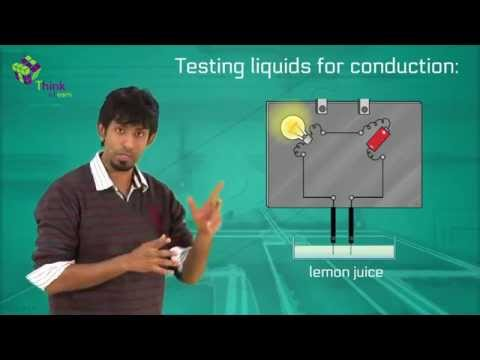# What are the applications of heating effect of electric current?

Applications of Heating effect of Electric current:

(i) Electrical fuse

(ii) Electric Heater (or) stove, Electric Radiator and electric iron

(iii) Electric welding and Electric furnace

## (i) Electrical fuse:

An electrical fuse is a short piece of wire with a low melting point, it is made of tin and lead alloy material.

• The fuse wire is connected in series with the electrical installation unit.
• When the electric current exceeds the rated value the fuse wire gets heated to a temperature higher than its melting point the wire is starting melting and the electrical circuit is cut off
• The temperature to which the wire is gets heated directly proportional to the square of the current and inversely proportional to the cube of the radius thus the temperature depends on the current flow through the wire and its radius irrespective of its length.

## (ii) Electric Heater (or) Electric stove, Electric radiator and Electric Iron:

All these are based on the principle of Joule’s heating effect heat will be produced in a wire when a steady current is possessed through it.

These electrical appliances contain a coil which is made up of an alloy of nicked and chromium when a large current in the order of 3 to 5 Amp can pass through them.

Example consider an Electrical Heater

An electrical heater is rated as 2.20 v and 1000 watts than the current through the coil will be

P = VI

$$\begin{array}{l}\frac{P}{V}=I\end{array}$$

$$\begin{array}{l}\frac{1000}{220}=I=4.55A\end{array}$$

Similarly resistance offered by the coil is

$$\begin{array}{l}P=\frac{{{v}^{2}}}{R}\end{array}$$

$$\begin{array}{l}R=\frac{{{v}^{2}}}{P}=\frac{{{\left( 220 \right)}^{2}}}{1000}=48.4 Omega\end{array}$$

The coil is placed along the axis of a parabolic metallic reflector (or) at the focus of a concave reflector.

## (iii) Electrical welding and Electric Furnace:

In electric welding

A strong electric current (i) is possessed through two metal bars when they are in contact the point of contact is made such a way to produce very high temperature in a short time by making very high resistance at the point of contact very high temperature is suitable for welding

Electric furnace

An electric furnace is made up of calcium carbide (i.e.m combination of calcium and carbon) and carborundum [combination of carbon and silicon] By applying a strong electric current through a coil of calcium carbide and carborundum are capable to produce various high temperatures.### Articles to Explore:(12)(5)